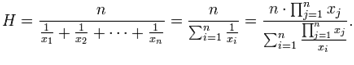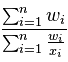Courses

Revision Notes - Harmonic Progression Notes | Study Mock Test Series for JEE Main & Advanced 2022 - JEE

JEE: Revision Notes - Harmonic Progression Notes | Study Mock Test Series for JEE Main & Advanced 2022 - JEE

The document Revision Notes - Harmonic Progression Notes | Study Mock Test Series for JEE Main & Advanced 2022 - JEE is a part of the JEE Course Mock Test Series for JEE Main & Advanced 2022.
All you need of JEE at this link: JEE
• Let a, b and c form an H.P. Then 1/a, 1/b and 1/c form an A.P.
• If a, b and c are in H.P. then 2/b = 1/a + 1/c,  which can be simplified as b = 2ac/(a+c)
• If ‘a’ and ‘b’ are two non-zero numbers then the sequence a, H, b is a H.P.
• The n numbers H1, H2, ……,Hn are said to be harmonic means between a and b, if a, H1, H2 ……, Hn, b are in H.P. i.e. if 1/a, 1/H1, 1/H2, ..., 1/Hn, 1/b are in A.P. Let d be the common difference of the A.P., Then 1/b = 1/a + (n+1) d ⇒ d = a–b/(n+1)ab.
Thus 1/H1 = 1/a + a–b/(n+1)ab,
1/H2 = 1/a + 2(a–n)/(n+1)ab,
………..     ……….
1/Hn = 1/a + n(a–b)/(n+1)ab.
• If x1, x2, … xare n non-zero numbers, then the harmonic mean ‘H’ of these numbers is given by 1/H = 1/n (1/x1 + 1/ x2 + ……. +1/ xn)• As the nth term of an A.P is given by an = a + (n-1)d, So the nth term of an H.P is given by 1/ [a + (n -1) d].
• If we have a set of weights w1, w2, …. , wn associated with the set of values x1, x2, …. , xn, then the weighted harmonic mean is defined• Questions on Harmonic Progression are generally solved by first converting them into those of Arithmetic Progression.
• If ‘a’ and ‘b’ are two positive real numbers then A.M x H.M = G.
• The relation between the three means is defined as A.M  >  G.M  >  H.M
• If we need to find three numbers in a H.P. then they should be assumed as 1/a–d, 1/a, 1/a+d
• Four convenient numbers in H.P. are 1/a–3d, 1/a–d, 1/a+d, 1/a+3d
• Five convenient numbers in H.P. are 1/a–2d, 1/a–d, 1/a, 1/a+d, 1/a+2d
The document Revision Notes - Harmonic Progression Notes | Study Mock Test Series for JEE Main & Advanced 2022 - JEE is a part of the JEE Course Mock Test Series for JEE Main & Advanced 2022.
All you need of JEE at this link: JEEUse Code STAYHOME200 and get INR 200 additional OFF Use Coupon Code

Top Courses for JEEMock Test Series for JEE Main & Advanced 2022

2 videos|258 docs|160 tests

Top Courses for JEETrack your progress, build streaks, highlight & save important lessons and more!

,

,

,

,

,

,

,

,

,

,

,

,

,

,

,

,

,

,

,

,

,

;